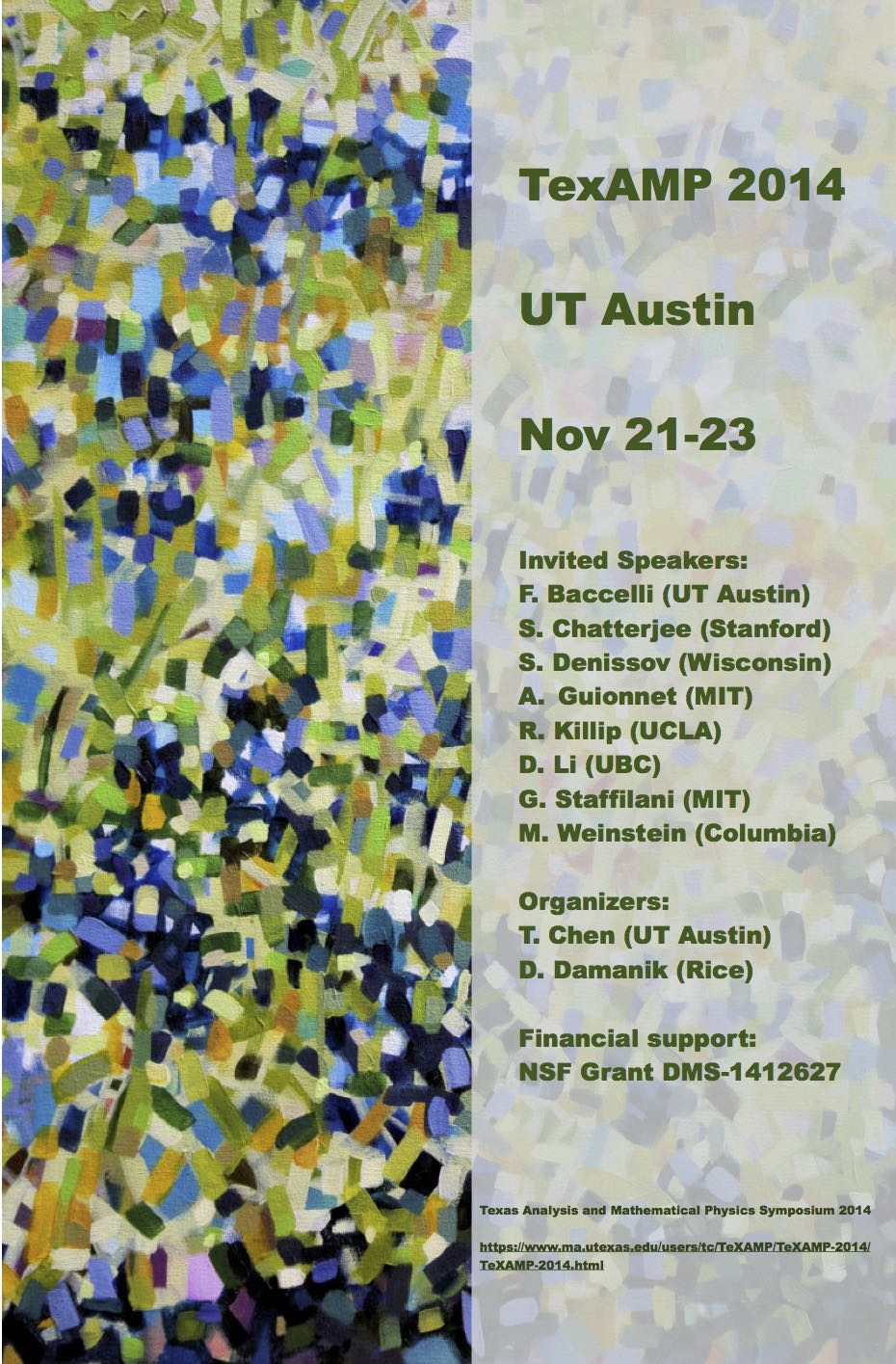# UT AUSTIN - Nov 21-23### Abstracts

 Francois Baccelli (University of Texas at Austin): Point-Map Probabilities of a Point Process A compatible point-shift \$f\$ maps, in a translation invariant way, each point of a stationary point process \$\Phi\$ to some point of \$\Phi\$. It is fully determined by its associated point-map, \$g^f\$, which gives the image of the origin by \$f\$. The initial question of this paper is whether there exist probability measures which are left invariant by the translation of \$-g^f\$. The point-map probabilities of \$\Phi\$ are defined from the action of the semigroup of point-map translations on the space of Palm probabilities, and more precisely from the compactification of the orbits of this semigroup action. If the point-map probability is uniquely defined, and if it satisfies certain continuity properties, it then provides a solution to the initial question. Point-map probabilities are shown to be a strict generalization of Palm probabilities: when the considered point-shift \$f\$ is bijective, the point-map probability of \$\Phi\$ boils down to the Palm probability of \$\Phi\$. When it is not bijective, there exist cases where the point-map probability of \$\Phi\$ is absolutely continuous with respect to its Palm probability, but there also exist cases where it is singular with respect to the latter. A criterium of existence of the point-map probabilities of a stationary point process is also provided. The results are illustrated by a few examples. Sourav Chatterjee (Stanford University): Invariant measures and the soliton resolution conjecture The soliton resolution conjecture for the focusing nonlinear Schrodinger equation (NLS) is the vaguely worded claim that a global solution of the NLS, for generic initial data, will eventually resolve into a radiation component that disperses like a linear solution, plus a localized component that behaves like a soliton or multi-soliton solution. Considered to be one of the fundamental problems in the area of nonlinear dispersive equations, this conjecture has eluded a proof or even a precise formulation till date. I will present a theorem that proves a "statistical version" of this conjecture at mass-subcritical nonlinearity. The proof involves a combination of techniques from large deviations, PDE, harmonic analysis and bare hands probability theory. Serguei Denissov (University of Wisconsin Madison): Contour dynamics for 2d Euler equation For the 2d Euler dynamics of patches, we will discuss two problems: merging of the central pair and the existence of the family of steady states. Alice Guionnet (MIT): Free probability and random matrices; from isomorphisms to universality Free probabiilty is a probability theory for non-commutative variables introduced by Voiculescu. It is equipped with a notion of freeness very similar to independence. It is a natural framework to study the limit of random matrices with size going to infinity. In this talk, we will discuss these connections and how they can be used to adapt ideas from classical probability theory to operator algebra and random matrices. We will in particular focus on how to adapt classical ideas on transport maps following Monge and Ampere to construct isomorphisms between algebras and prove universality in matrix models. This talk is based on joint works with F. Bekerman, A. Dabrowski, A. Figalli, E. Maurel-Segala, J. Novak, and D. Shlyakhtenko. Rowan Killip (UCLA): Strichartz estimates on tori Dong Li (UBC): Norm inflation for incompressible Euler We will discuss some recent progress on understanding the norm inflation and illposedness of solutions to the incomressible Euler equations in borderline spaces. (Joint work with Jean Bourgain). Gigliola Staffilani (MIT): Randomization and existence of large data solutions at critical and supercritical regimes In this talk I will consider some initial value problems that are critical or supercritical with respect to the regularity of the data. For these problems I will summarize some of the results concerning with existence of large data solutions for long time obtained by using randomization. I will also present a recent result involving almost sure global well-posedness and the non-squeezing theorem. (Most of the work presented is in collaboration with Andrea Nahmod. The work on the non-squeezing theorem is by my student Dana Mendelson.) Michael I. Weinstein (Columbia University): Waves in Honeycomb Structures I will discuss the propagation of waves in honeycomb-structured media. The (Floquet-Bloch) dispersion relations of such structures have conical singularities which occur at the intersections of spectral bands for high-symmetry quasi-momenta. These conical singularities, also called Dirac points or diabolical points, are central to the remarkable electronic properties of graphene and the light-propagation properties in honeycomb structured dielectric media. Examples of such properties are: quasi-particles which behave as massless Dirac Fermions, tunability between conducting and insulating states and topologically protected edge states. Most theoretical work on honeycomb structures (going back to 1947) has centered on the tight-binding approximation, a solvable discrete limit, corresponding to infinite medium contrast. I will first discuss results (with C.L. Fefferman) on the dispersive wave properties of the Schroedinger equation with a honeycomb lattice potential in which no assumptions are made on the size of the potential (medium contrast). I’ll next discuss results (with C.L. Fefferman and J.P. Lee-Thorp) on a 1D dimer model with topologically protected edge states, and then work in progress on edge states in 2D honeycomb structures. Return to main page